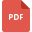# decimals and division tutorialsMultiplying and Dividing Decimals
Mathematics, Grade 7, United States, Decimals and Division, Decimals and Multiplication, Decimal and FractionsMultiplying and Dividing decimals by 10, 100, and 1000
Decimals and Multiplication, Grade 5, United States, Decimals and Division